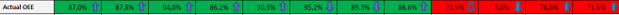# QlikView Scripting

Discussion Board for collaboration on QlikView Scripting.

Honored Contributor III

## Weekly , Monthly , Yearly Compare Help

Hi Folks,

I'm having below expression:

OEE=

if(sum([Act Planned Production Hours]) = 0, avg([Act OEE]),

sum([Act OEE]*[Act Planned Production Hours])/sum([Act Planned Production Hours]))

Now value of above exprssion I have to compared based on last week , last month , last Year

For example :

if (Week 38 value of OEE is > last week i.e . 37 ,'▲','▼')

similarly if (Sep value of OEE > Aug Value of OEE,'▲','▼')

and if (2016 OEE > 2015 OEE ,'▲','▼')

Result should be something like below:Any Suggestions???

Thanks,

AS

10 Replies
Highlighted
New Contributor III

## Re: Weekly , Monthly , Yearly Compare Help

Hi,

you can use a table wiht as many meassures as needed. In each meassure you have to use set analysis to fix the current week and the compared week.

Regards,

GabrielPartner

## Re: Weekly , Monthly , Yearly Compare Help

Hi Amit,

Try with below expression for

(2016 OEE > 2015 OEE ,'▲','▼')

if(

(if(sum({<Year = {"\$(=max(Year))"}>} [Act Planned Production Hours]) = 0, avg({<Year = {"\$(=max(Year))"}>} [Act OEE]),

sum({<Year = {"\$(=max(Year))"}>} [Act OEE]*[Act Planned Production Hours])/sum({<Year = {"\$(=max(Year))"}>} [Act Planned Production Hours])))

>

(if(sum({<Year = {"\$(=max(Year)-1)"}>} [Act Planned Production Hours]) = 0, avg({<Year = {"\$(=max(Year)-1)"}>} [Act OEE]),

sum({<Year = {"\$(=max(Year)-1)"}>} [Act OEE]*[Act Planned Production Hours])/sum({<Year = {"\$(=max(Year)-1)"}>} [Act Planned Production Hours])))

,1,0)

For Month and Week comparison, just change set analysis.

Regards,

Valued Contributor

## Re: Weekly , Monthly , Yearly Compare Help

I'm not very sure,

But you can try something like this:

Example: for Month--->. if (Sep value of OEE > Aug Value of OEE,'▲','▼')

1. You can make a straight table

2. Dimensions: YearMonth (eg: 201607) , Your_Field1

3. Expression: Create a Month over Month comparison ---> eg: sum((Sale_Amount)/Convert)

Growth --- diff of two month using above()  --->

eg: =(sum((Sale_Amount)/Convert)-above(sum((Sale_Amount)/Convert)))/above(sum((Sale_Amount)/Convert))

4. Hide everything and just keep Growth expression.

5. Also, in presentation check horizontal.

6. Then you can format everything, like giving background colors and all.

I'm not sure.

Valued Contributor

## Re: Weekly , Monthly , Yearly Compare Help

I think , I'm missing something.

Valued Contributor

## Re: Weekly , Monthly , Yearly Compare Help

May be like this:MVP

## Re: Weekly , Monthly , Yearly Compare Help

May be use set analysis to get previous week, month and year values to do a comparison:

The Magic of Set Analysis - Point In Time Reporting • Blog • AfterSync

Honored Contributor III

## Re: Weekly , Monthly , Yearly Compare Help

Thanks for the suggestion , could u plz check why this is not working for Week comparison:

if(

(if(sum({<Week = {"\$(=max(Week))"},Year_KPI=,POPER_NEW=,Week=>} [Act Planned Production Hours]) = 0, avg({<Week = {"\$(=max(Week))"},Year_KPI=,POPER_NEW=,Week=>} [Act OEE]),

sum({<Week = {"\$(=max(Week))"},Year_KPI=,POPER_NEW=,Week=>} [Act OEE]*[Act Planned Production Hours])/sum({<Week = {"\$(=max(Week))"},Year_KPI=,POPER_NEW=,Week=>} [Act Planned Production Hours])))

>

(if(sum({<Week = {"\$(=max(Week)-1)"},Year_KPI=,POPER_NEW=,Week=>} [Act Planned Production Hours]) = 0, avg({<Week = {"\$(=max(Week)-1)"},Year_KPI=,POPER_NEW=,Week=>} [Act OEE]),

sum({<Week = {"\$(=max(Week)-1)"},Year_KPI=,POPER_NEW=,Week=>} [Act OEE]*[Act Planned Production Hours])/sum({<Week = {"\$(=max(Week)-1)"},Year_KPI=,POPER_NEW=,Week=>} [Act Planned Production Hours]))),

'▲' &num((if(sum([Act Planned Production Hours]) = 0, avg([Act OEE]),

sum([Act OEE]*[Act Planned Production Hours])/sum([Act Planned Production Hours]))

),'#.##0,0%')

,'▼' & num((if(sum([Act Planned Production Hours]) = 0, avg([Act OEE]),

sum([Act OEE]*[Act Planned Production Hours])/sum([Act Planned Production Hours]))

),'#.##0,0%'))

Thanks,

ASPartner

## Re: Weekly , Monthly , Yearly Compare Help

Hi amit,

Add year aslo in set analysis. If week comparision for current year then you have to mention it in set analysis.

Else it will compare week 2 value of all years with week 1 value of all years.

To avoid that you will have to specify which year to consider for week comparision.

You will have to do same for month comparision.

in your expression, you have nulified week also. you will have to remove that.

if(

(if(sum({<Week = {"\$(=max(Week))"},Year_KPI=,POPER_NEW=,Week=>} [Act Planned Production Hours]) = 0, avg({<Week = {"\$(=max(Week))"},Year_KPI=,POPER_NEW=,Week=>} [Act OEE]),

sum({<Week = {"\$(=max(Week))"},Year_KPI=,POPER_NEW=,Week=>} [Act OEE]*[Act Planned Production Hours])/sum({<Week = {"\$(=max(Week))"},Year_KPI=,POPER_NEW=,Week=>} [Act Planned Production Hours])))

>

(if(sum({<Week = {"\$(=max(Week)-1)"},Year_KPI=,POPER_NEW=,Week=>} [Act Planned Production Hours]) = 0, avg({<Week = {"\$(=max(Week)-1)"},Year_KPI=,POPER_NEW=,Week=>} [Act OEE]),

sum({<Week = {"\$(=max(Week)-1)"},Year_KPI=,POPER_NEW=,Week=>} [Act OEE]*[Act Planned Production Hours])/sum({<Week = {"\$(=max(Week)-1)"},Year_KPI=,POPER_NEW=,Week=>} [Act Planned Production Hours]))),

'▲' &num((if(sum([Act Planned Production Hours]) = 0, avg([Act OEE]),

sum([Act OEE]*[Act Planned Production Hours])/sum([Act Planned Production Hours]))

),'#.##0,0%')

,'▼' & num((if(sum([Act Planned Production Hours]) = 0, avg([Act OEE]),

sum([Act OEE]*[Act Planned Production Hours])/sum([Act Planned Production Hours]))

),'#.##0,0%'))

Regards,

Honored Contributor III

## Re: Weekly , Monthly , Yearly Compare Help

Even tried something below for Week comparison , but not working:

=if(

(if(sum({\$<%DATE_Key={">=\$(=WeekStart(today()))<=\$(=WeekEnd(today()))"}>}[Act Planned Production Hours]) = 0, avg({\$<%DATE_Key={">=\$(=WeekStart(today()))<=\$(=WeekEnd(today()))"}>}[Act OEE]),

sum({\$<%DATE_Key={">=\$(=WeekStart(today()))<=\$(=WeekEnd(today()))"}>}[Act OEE]*[Act Planned Production Hours])/sum({\$<%DATE_Key={">=\$(=WeekStart(today()))<=\$(=WeekEnd(today()))"}>}[Act Planned Production Hours])))

>

(if(sum({\$<%DATE_Key={">=\$(=WeekStart(today()-7))<=\$(=WeekEnd(today()-7))"}>}[Act Planned Production Hours]) = 0, avg({\$<%DATE_Key={">=\$(=WeekStart(today()-7))<=\$(=WeekEnd(today()-7))"}>}[Act OEE]),

sum({\$<%DATE_Key={">=\$(=WeekStart(today()-7))<=\$(=WeekEnd(today()-7))"}>}[Act OEE]*[Act Planned Production Hours])/sum({\$<%DATE_Key={">=\$(=WeekStart(today()-7))<=\$(=WeekEnd(today()-7))"}>}[Act Planned Production Hours])))

,

'▲' &num((if(sum([Act Planned Production Hours]) = 0, avg([Act OEE]),

sum([Act OEE]*[Act Planned Production Hours])/sum([Act Planned Production Hours]))

),'#.##0,0%')

,'▼' & num((if(sum([Act Planned Production Hours]) = 0, avg([Act OEE]),

sum([Act OEE]*[Act Planned Production Hours])/sum([Act Planned Production Hours]))

),'#.##0,0%'))

Thanks,
AS